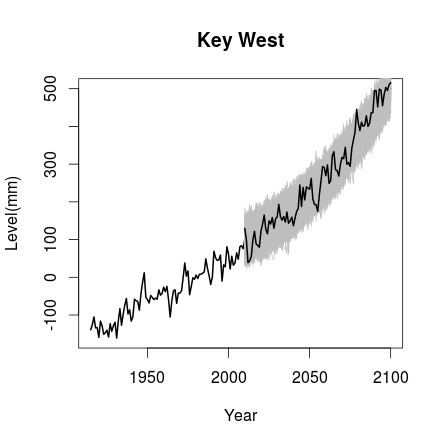# Timescales for detecting SLR acceleration

Recent interest in a paper on sea level rise acceleration (Haigh et al. 2014) has led me to attempt to reproduce their analysis.

Below is R code that I wrote to examine one of the stations from the paper (Key West). In future posts I will expand on this code to check the “sliding” window portion of the paper.##obtain annual mean sea level for Key West from:
#http://www.psmsl.org/data/obtaining/rlr.annual.data/188.rlrdata

names(dt)<-c("date","level")
dt<-dt[3:97,] #restrict date range to same as reference 1915-2009
dt[39,2]<-(dt[38,2]+dt[40,2])/2 #fill missing value at 1953 by linear interpolation
dt[,2]<-dt[,2]-min(dt[,2])#center series

linearfit<-lm(dt$level~dt$date)
dt$detrend<-dt$level-(linearfit$coefficients*dt$date)
ar1fit<-arima(dt$detrend,order=c(1,0,0)) #fit ar1 lm(dt$level~poly(dt$date,2,raw=T))$coefficients*2 #check acceleration estimate from table 2

#create ar5 mid projection of 0.5 m (500 mm) incease from 1990 to 2100
x<-seq(from=0,to=109,by=1)
y<-x^2*8.25+x*169.25+dt[95,2]#smooth quadratric not from IPCC
a<-(500-76)/y[length(y)]#scaling factor
y2<-y[20:110]*a+76
level2<-append(dt$level-dt[76,2],y2)#down-shift time series to match haigh (level is 0 at 1990) level2<-cbind(data.frame(seq(from=1915,to=2100,by=1)),data.frame(level2)) names(level2)<-c("year","level") plot(level2$year,level2$level,type="l",main="Key West",ylab="Level(mm)",xlab="Year",lwd=2) #simulate future oscillations from ar1fit for(i in 1:1000){ ar.sim<-arima.sim(list(ar=c(as.numeric(ar1fit$coef))),n=length(y2),sd=23)#sd value from haigh et al not able to reproduce
lines(seq(from=2010,to=2100,by=1),y2+ar.sim,col="grey")
}
lines(seq(from=2010,to=2100,by=1),y2+ar.sim,lwd=2)


###Reference > I. D. Haigh, T. Wahl, E. J. Rohling, R. M. Price, C. B. Pattiaratchi, F. M. Calafat, S.Dangendorf, “Timescales for detecting a significant acceleration in sea level rise”, Nature Communications 5, Article number: 3635, http://dx.doi.org/10.1038/ncomms4635, published 14 April 2014. >